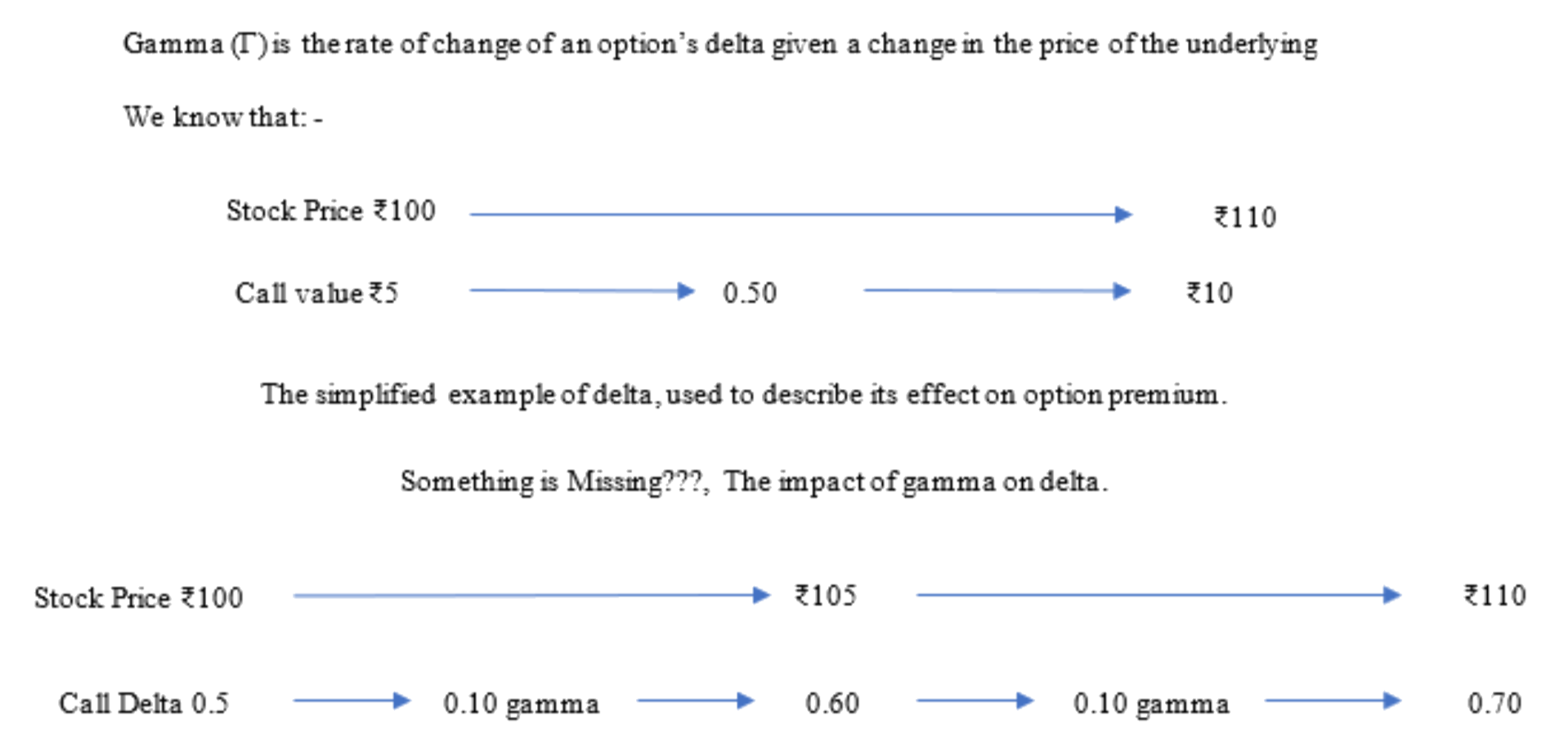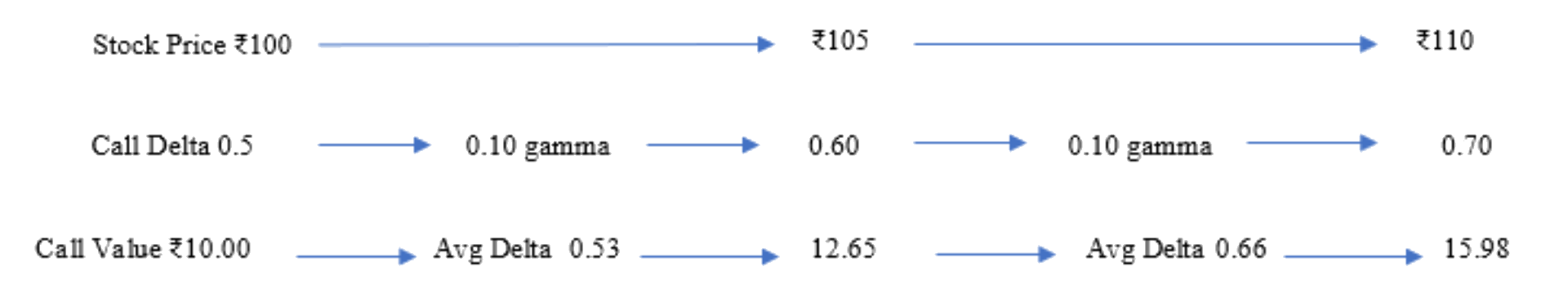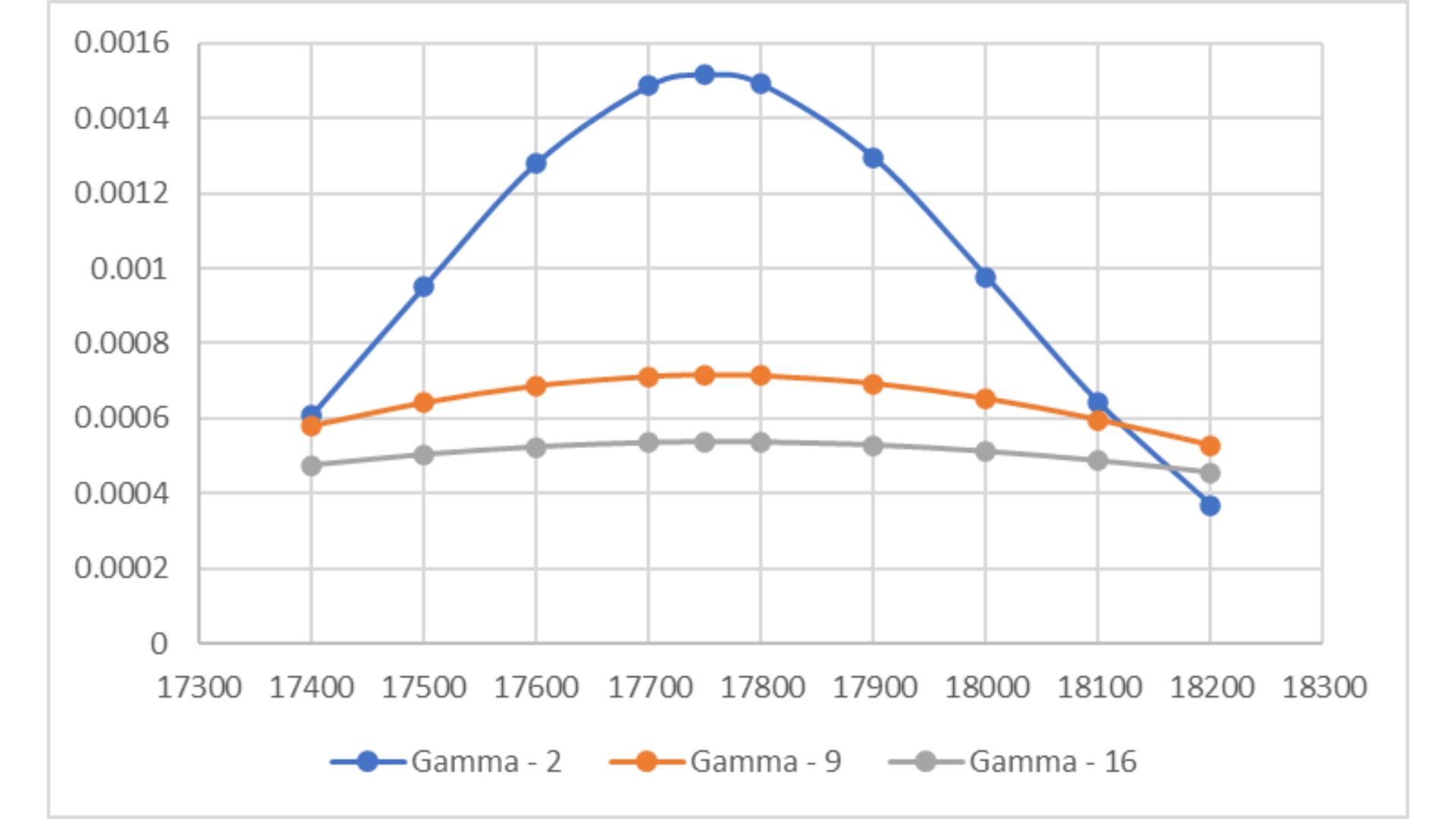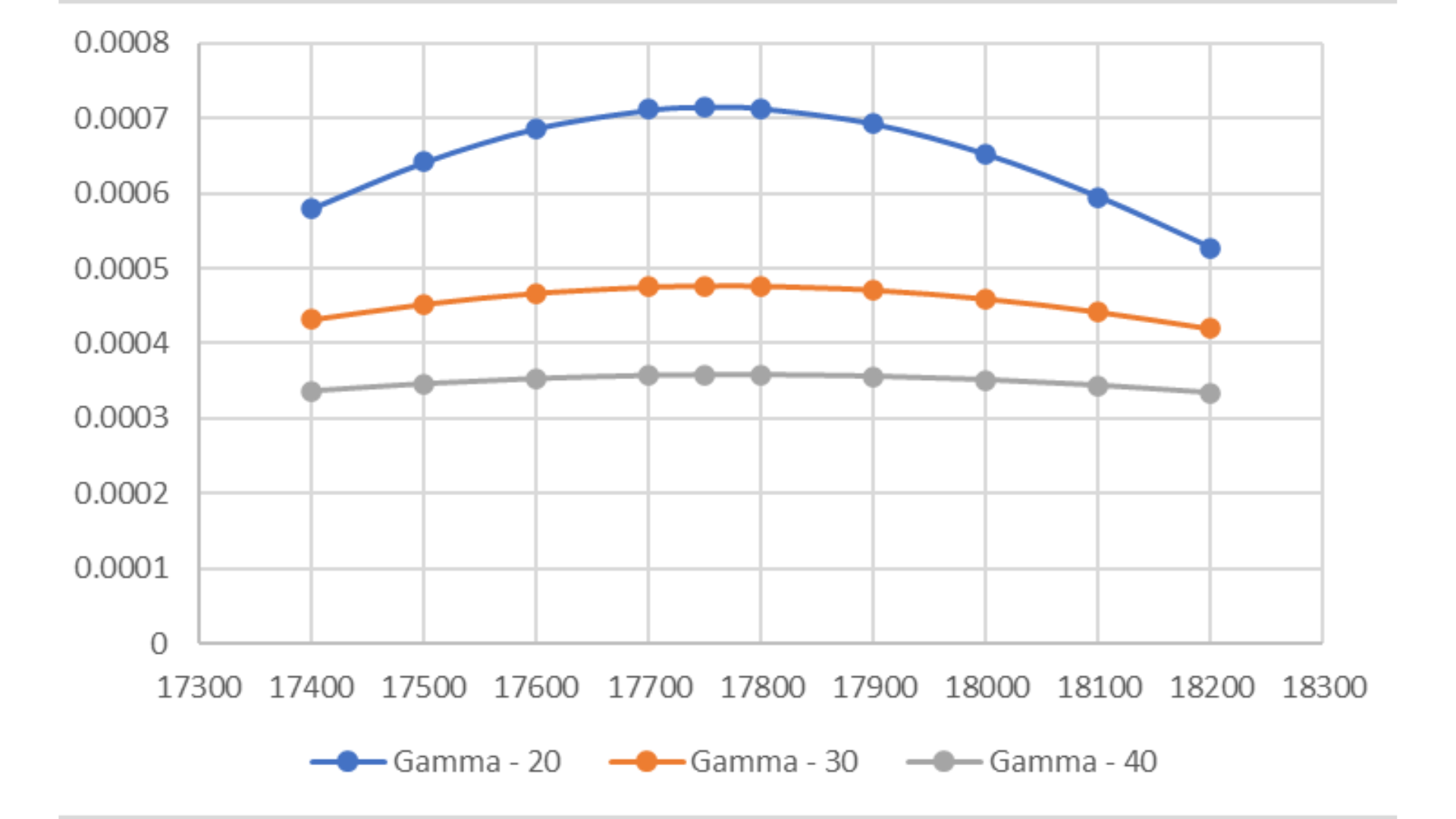GammaGamma

# Gamma

Gamma is a second-order Greek in options trading and refers to the rate of change of an option's delta per unit change in the underlying asset's price.

Gamma is a crucial Greek to understand when managing an options portfolio, as it helps traders anticipate the potential impact that changes in the price of the underlying asset will have on the option's delta and, as a result, its potential profitability. A positive gamma means that an option's delta will increase as the underlying asset's price rises, and decrease as the underlying asset's price falls. A negative gamma means the opposite, with the option's delta decreasing as the underlying asset's price rises and increasing as the underlying asset's price falls.

## Example of GAMMAThe call in this example starts as a 0.50-delta option. When the stock price increases by Rs 5.00, the delta increases by the amount of the gamma. In this example, delta increases from 0.50 to 0.60, adding 0.10 deltas. As the stock price continues to rise, the delta continues to move higher. At Rs110, the call’s delta is 0.70.

This increase in delta will affect the value of the call. When the stock price first begins to rise from Rs100, the option value is increasing at a rate of 50 percent—the call’s delta at that stock price. But by the time the stock is at Rs105, the option value is increasing at a rate of 0.60 percent of the stock price.

In terms of option variables, gamma is affected by a number of factors, including the time to expiration, the price of the underlying asset, the strike price, the implied volatility, and the interest rate.The above example elucidates, how the changing delta, due to jump in underlying can impact or influence option premium. The assumed average delta during the first jump of Rs 5 in the stock price is about 0.53 and in the second  jump is about 0.66.

As the time to expiration decreases, the option's gamma increases. This is because the option's delta becomes more sensitive to changes in the underlying asset's price as it approaches expiration. Similarly, as the price of the underlying asset approaches the strike price, the option's gamma increases, as the option's delta becomes more sensitive to changes in the price of the underlying asset.

A gamma explosion is a term used to describe a rapid increase in the rate of change of an option's delta (the sensitivity of the option's price to the underlying asset's price) as the option approaches its expiration date. This can occur when there is a large increase in the underlying asset's price and can result in large losses for option sellers who have not properly managed their delta exposure.

It is important for option traders to understand the potential for gamma explosion and to manage their delta exposure through proper option hedging techniques. This can help to minimize the potential for large losses and ensure that the trader's portfolio is better protected against market volatility.

## Characteristics of Option Greek, Gamma

• Gamma is highest for ATM options. In general, the option greeks gamma risk of an option position is highest for at-the-money options and decreases as the options move further in- or out-of-the-money.

•

Gamma increases as days to expiry (DTE) decrease ---- Gamma explosion.

•

Gamma decreases as volatility of the underlying, namely, Nifty/BankNifty or F&O stocks on NSE, increases.The above chart indicates gamma explosion, i.e. as DTE decreases, the option greeks gamma of Nifty options increases. Gamma – 2 is with DTE =2, for Nifty options with ATM = 17750, volatility at 20%.The above chart indicates impact of volatility on gamma option, i.e. as volatility increases, the gamma of Nifty options decreases and the curve flattens. Gamma – 2 series, is with DTE =2, for Nifty options with ATM = 17750, volatility at 20%.The above chart indicates impact of volatility on gamma, i.e. as volatility increases, the gamma of Nifty options decreases and the curve flattens. Gamma – 2 series, is with DTE =2, for Nifty options with ATM = 17750, volatility at 40%.

### What is option gamma risk?

Gamma is an important option Greek, to consider, for option traders because it helps them understand the potential risks involved with a particular trade. For example, a high gamma option position means that the delta will change significantly for even small moves in the underlying asset, which can result in large losses if the market moves against the option trader.

### Which options have the highest gamma?

Gamma is highest for ATM options. In general, the gamma risk of an option position is highest for at-the-money options and decreases as the options move further in- or out-of-the-money.

### Should gamma be high or low in options?

It depends on the risk-profile of the option trader. For conservative traders, who prefer limited risk, low gamma is usually desirable. This means that the delta of the option position will change relatively slowly for small movements in the underlying asset's price, with the underlying being Nifty/BankNifty/FinNifty or F&O stocks. This reduces the potential for large losses if the market moves against the trader.

On the other hand, traders who are comfortable with higher risk and are looking to take more aggressive trades may prefer high gamma positions. These options are more sensitive to changes in the underlying asset's price, allowing for the potential for larger profits if the market moves in the right direction, while losses are also magnified, if markets move adversely.